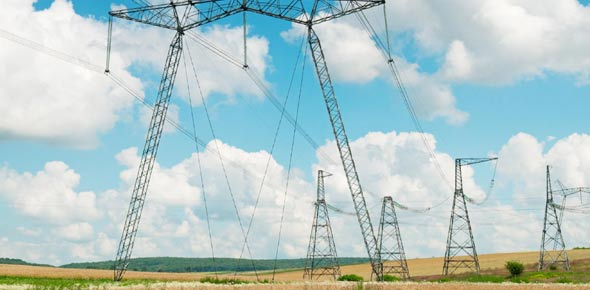# Aes Units Of Electricity Quiz

Approved & Edited by ProProfs Editorial Team
At ProProfs Quizzes, our dedicated in-house team of experts takes pride in their work. With a sharp eye for detail, they meticulously review each quiz. This ensures that every quiz, taken by over 100 million users, meets our standards of accuracy, clarity, and engagement.
| Written by Bsp210
B
Bsp210
Community Contributor
Quizzes Created: 1 | Total Attempts: 128
Questions: 8 | Attempts: 128SettingsQuiz created for use of year 9 KS3 pupils at the Anglo European School. The quiz covers aspects of electrical power ratings and energy consumption.

• 1.

### Most large pieces of electrical equipment in our homes use electricity from the mains supply.What is the voltage of the mains supply?

• A.

220V

• B.

230V

• C.

240V

• D.

250V

• E.

12V

B. 230V
Explanation
The mains voltage entering your house in the UK is 230V.

Rate this question:

• 2.

### Some appliances use more energy than others. Electrical fires use 1000J of electrical energy every second where radios use 100J per second.What would use more energy; listening to the radio for 40 minutes or using an electrical fire for 15 minutes?

• A.

The radio would use more electrical energy

• B.

The electric fire would use more energy

• C.

Both items would use the same amount of energy

B. The electric fire would use more energy
Explanation
The electrical fire uses 1000J of energy every second, there are 900 seconds in 15 minutes so the total energy used is equal to 900 seconds multiplyed by 1000 joules:
900*1000=900,000 J
The electrical fire uses 900,000 joules in 15 minutes.

The radio uses 100 joules per second for 40 minutes. There are 2400 seconds in 40 minutes. The total energy used by the radio is equal to:
2400 seconds * 100J = 240,000J
The radio less energy (240,000J) in 40 minutes than electrical fire does in 15 minutes (900,000J).

Rate this question:

• 3.

### If I run an electrical fire for 15 minutes it uses 900,000 joules of energy. How many minutes would I have to listen to a radio for the use this much energy?The radio uses 100 joules of electrical energy per second.

• A.

100 minutes

• B.

150 minutes

• C.

225 minutes

• D.

1000 minutes

• E.

1500 minutes

B. 150 minutes
Explanation
The fire used 900,000 joules. The radio uses 100 joules per second. First work out how many seconds we would need to use the radio for to use 900,000 J:
900,000J / 100J = 9,000 seconds
So we need to use the radio for 9000 seconds to require the same amount of energy. The answer needs to be in minutes so to convert seconds to minutes we divide by 60:
9000 seconds / 60 = 150 minutes

Therefore, we need to listen to the radio for 150 minutes to use the same amount of energy as an electric fire uses in 15 minutes.

Rate this question:

• 4.

### The energy we use has to be paid for. The more energy we use the ___________ the electricity bill. We can save money by only using electrical equipment when we need it.

bigger
higher
more
more expensive
Explanation
Like most things we use we have to pay for electricity. The more electricity we use the more we are charged. Power companies measure how much electricity we use on an electric meter.

Rate this question:

• 5.

### We can find out how much energy differnet appliances use by looking at their power rating. The power of an appliance is the number of joules of energy it uses each second.What are the units of power?

• A.

Ohms

• B.

Hertz (HZ)

• C.

Watts (W)

• D.

Centimeters (CM)

C. Watts (W)
Explanation
Power is measured in Watts

Rate this question:

• 6.

### ______ watt(s)= 1 joule used every second

one
1
Explanation
Watts are the unit of power. The power of an appliance describes the rate at which the appliance uses energy. Power measured in Watts refers to how much energy (joules) an appliance uses each second. An appliance that uses 1 joule of energy per second has a power of 1 Watt.

Rate this question:

• 7.

### A toaster has a power rating of 1100W. It takes 1 minute (60 seconds) to make taost in it. How many joules of energy are required to make a slice of toast?

• A.

660

• B.

6500

• C.

66000

• D.

650000

C. 66000
Explanation
The toaster has a power rating of 1100W this means it uses 1100 joules per second (1 joules = 1 Watt). It takes 60 seconds to make toast and 1100 J are used every second so:

60 seconds x 1100J =66000 J

66,000 joules are required

Rate this question:

• 8.

### When appliances have a high power rating the power is often given in Kilowatts (kW). If a kettle has a power rating of 2250 Watts how would it be expressed as Kilowatts?

• A.

0.225 kW

• B.

2.25 kW

• C.

22.5 kW

• D.

225 kWBack to top